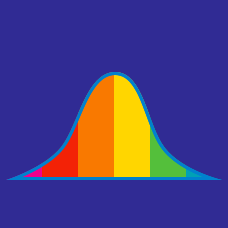Probability

# Data - Range

For a test which is scored out of 100, half of the students scored 70 and the other half of the students scored 81. What is the range of their scores?

$\{ 4, 7, 10, 18, 26 \}$

What is the range of the above set?

$\begin{array}{c}&-14, &21, &-17, &25, &0, &-19, &11, &-20, &18\end{array}$

The above are the points by which Jack's basketball team won/lost against his rival team in the last 9 games. What is the range of these points?

$\{ -3, 7, 10, -25, 35 \}$

What is the range of the above set?

10 students took a test that was scored out of 10.
1 of them got a score of 3,
3 of them got a score of 5,
and 6 of them got a score of 7.

What is the range of scores?

×